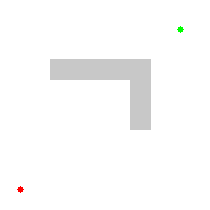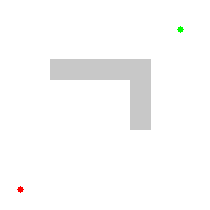f=g+h is an medium difficulty programming/misc challenge from YASCON 2020, in this blog we’ll be discussing the intended way to solve the challenge.

##### Introduction:

We’re given an archive with a word map and a text file with a maze in it. From the text file description and the challenge name, it’s clear that we have to implement A* pathfinding algorithm and find the fastest route to the element X.

Pathfinding is basically plotting the shortest route between two points. It is a more practical variant on solving mazes. This field of research is based heavily on Dijkstra’s algorithm for finding the shortest path on a weighted graph. Here we’re going to use an extension of Dijkstra’s algorithm called A* because it achieves better performance by using heuristics to guide its search.Dijkstra's AlgorithmA* Algorithm

##### A* Algorithm:

A* assigns a weight to each open node equal to the weight of the edge to that node plus the approximate distance between that node and the finish. This approximate distance is found by the heuristic and represents a minimum possible distance between that node and the end. This allows it to eliminate longer paths once an initial path is found.

One important aspect of A* is `f = g + h`. The f, g and h variables are in our Node class and get calculated every time we create a new node. Quickly I’ll go over what these variables mean.

• F is the total cost of the node.
• G is the distance between the current node and the start node.
• H is the heuristic — estimated distance from the current node to the end node.

We won’t be implementing A* ourselves, as it’s a waste of time to reinvent the wheel, so instead we’ll be using someone else’s code 😅
Here’s a python implementation of A* from scratch: gist. All thanks to ryancollingwood 🙌Change the maze on line 137 with the maze given in the challenge file, Set the destination value to coordinates of the element ‘X’, in our case (7, 8). So the script starts finding the shortest path from (0,0) to (7,8).After getting the coordinates, we need to use them on the image and map out its respective character. All we have to do is just figure out what character stands in the path coordinates to find the flag. You can do that in many ways or even manually. I have created a script to visualize it using Matplotlib and OpenCV, it basically creates a table from the image and plots the points using the coordinates.And we get the string - AESKDCDOHKKLAOPOLGTMLOME
Since the flag is MD5Sum of the path string,

YASCON{45131ca9f5140debc67047351d21a403}

If you wanted to try it for yourself, here’s the challenge files: chall.zip ✌️